4186 görüntülenme
Quad-HullMA-cross & VWMA & MacD & TSI combination

Version 4.20

calc_on_order_fills= true, calc_on_every_tick=true, default_qty_type=strategy.percent_of_equity, default_qty_value=100, pyramiding=0
```//@version=2
//                                                    Quad-HullMA-cross & VWMA & MacD & TSI combination  <<<<< by SeaSide420 >>>>>>
strategy("MultiCross420", overlay=true, calc_on_order_fills= true, calc_on_every_tick=true, default_qty_type=strategy.percent_of_equity, default_qty_value=100, pyramiding=0)
keh=input(title="Double HullMA 1",type=integer,defval=7, minval=1)
teh=input(title="Double HullMA 2",type=integer,defval=14, minval=1)
meh=input(title="VWMA",type=integer,defval=1, minval=1)
meh1=vwma(close,round(meh))
n2ma=2*wma(close,round(keh/2))
nma=wma(close,keh)
diff=n2ma-nma,sqn=round(sqrt(keh))
n2ma1=2*wma(close,round(keh/2))
nma1=wma(close,keh)
diff1=n2ma1-nma1,sqn1=round(sqrt(keh))
n1=wma(diff,sqn)
n2=wma(diff1,sqn)
b=n1>n2?lime:red
c=n1>n2?green:red
n2ma3=2*wma(close,round(teh/2))
nma2=wma(close,teh)
diff2=n2ma3-nma2,sqn2=round(sqrt(teh))
n2ma4=2*wma(close,round(teh/2))
nma3=wma(close,teh)
diff3=n2ma4-nma3,sqn3=round(sqrt(teh))
n3=wma(diff2,sqn2)
n4=wma(diff3,sqn3)
fastLength = input(title="MacD fastLength", type=integer,defval=7)
slowlength = input(title="MacD slowlength", type=integer,defval=14)
MACDLength = input(title="MacD Length", type=integer,defval=3)
MACD = ema(close, fastLength) - ema(close, slowlength)
aMACD = ema(MACD, MACDLength)
delta = MACD - aMACD
a1=plot(n1,color=c),a2=plot(n2,color=c)
plot(cross(n1, n2) ? n1 : na, style = cross, color=b, linewidth = 3)
a3=plot(n3,color=c),a4=plot(n4,color=c)
plot(cross(n3, n4) ? n1 : na, style = cross, color=b, linewidth = 3)
//a5=plot(meh1,color=c)
long = input(title="TSI Long Length", type=integer, defval=5)
short = input(title="TSI Short Length", type=integer, defval=3)
signal = input(title="TSI Signal Length", type=integer, defval=2)
linebuy = input(title="TSI Upper Line", type=integer, defval=4)
linesell = input(title="TSI Lower Line", type=integer, defval=-4)
price = close
double_smooth(src, long, short) =>
fist_smooth = ema(src, long)
ema(fist_smooth, short)
pc = change(price)
double_smoothed_pc = double_smooth(pc, long, short)
double_smoothed_abs_pc = double_smooth(abs(pc), long, short)
tsi_value = 100 * (double_smoothed_pc / double_smoothed_abs_pc)
closelong = n1<n2 and n3<n4 and n1>meh1
if (closelong)
strategy.close("Long")
closeshort = n1>n2 and n3>n4 and n1<meh1
if (closeshort)
strategy.close("Short")
longCondition = strategy.opentrades<1 and n1>n2 and MACD>aMACD and n1<meh1 and n3>n4 and ema(tsi_value, signal)>linesell
if (longCondition)
strategy.entry("Long",strategy.long)
shortCondition = strategy.opentrades<1  and n1<n2 and MACD<aMACD and n1>meh1 and n3<n4 and ema(tsi_value, signal)<linebuy
if (shortCondition)
strategy.entry("Short",strategy.short)```

## Yorumlar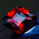Hi

Is there an updated version of this script (MultiCross v4.20
Jun 10, 2016) or a better one you have done?

Looking for a HIGH Profit Percentage on a MA cross script mixed with other indicators.

Thanks
Cevap Gönder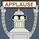I really like this script but it would be awesome if one could do alerts with it as well which it doesn't seem to do.
Cevap Gönder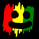MagnusSoderberg
@MagnusSoderberg, its quite easy to convert this into a Study (instead of a strategy) then alerts will work.
Cevap Gönder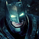@SeaSide420 Nice work! One question: Does strategy repaint? Tks!
Cevap GönderAllMountain
@AllMountain, yes.
it needs to be edited.
everywhere it says ""close"", change to a ""ohlc4""
Cevap GönderSeaSide420
@SeaSide420, ohlc4 but change the things to brackets. this website not allow to type brackets
Cevap Gönder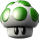SeaSide420
@SeaSide420,
Hi,
I do not understand what you said.
Could you post a picture of the code of "close -> ohlc4"?
I would appreciate if you can.
Cevap Gönder